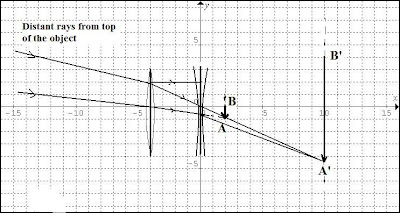## Saturday, June 25, 2011

### Solutions to compound lens problemsProblems from Pt. 20:

(1) A telephoto lens consists of a converging lens of focal length 6 cm placed 4 cm in front of a diverging lens of focal length (-2.5 cm).

a) Do a graphical construction of the system showing where the image would be.
b) Compare the size of the image formed by this combination with the size of the image that would be formed by the positive lens alone.
-----------

Solutions:

a) The graphical constuction is shown in the accompanying diagram, showing the image is 10 cm from the optical axis of the diverging lens. If the negative (diverging ) lens had not been used then the image AB would have been formed at the principal focal plane of the +6 cm (converging) lens, 6 cm from it. However, the diverging lens decreases the convergence of the rays (left side) refracted by the converging lens and causes them to focus at A'B', 14 cm from the converging lens - and 10cm from the diverging lens, as shown.

b) The image AB that would have been formed by the converging lens alone is (6 cm - 4 cm) = 2 cm beyond the f = (-2.5 cm) lens and is taken as the virtual object for that lens. ThenL s1 = -2 cm, and:

1/s1' = 1/f - 1/s1 = 1/ (-2.5) - 1/(-2) = -1/2.5 + 1/2 = 1/10

Then: s1' = 10 cm

Thus, the final image A'B' is real and 10 cm beyond the divergin lens - as the graphical construction shows.

The linear magnification: M1 = (-s1'/ s1) = (10 cm/ 2 cm) = 5

and since, h'/h = 5, then h' = 5h so the image formed by the combination is 5x larger than that formed by the (+) lens alone.

2) The objective lens of an astronomical telescope has a focal length of 6 ft. The eyepiece has a focal length of 2 inches.

a) Find the angular magnification that the telescope will produce when used for distant objects.

b) A rule for observing extended astronomical objects, such as planets or nebulae, is that the telescope magnification should not exceed 60x per inch of objective aperture.
----

Solutions:

(a) M = F/ f(e) where F = 6' = 72" and f(e) = 2"

Then: M = 72"/ 2" = 36 x

(b) It is not possible to strictly assert the condition is met (since no aperture is provided) but given the long focal length (6') it is more likley the aperture is at least 6" so the condition is easily met. (60x per in. would be 360x. Even a 1" aperture would easily meet the condition, however.)

If the astronomical telescope of this problem is used to observe the planet Jupiter,is the condition met or not? If not, what focal length eyepiece is needed to get the maximum angular magnification?
---

Soln.

Assuming a 6" aperture to get 360x for Jupiter then we'd need: M= 360 and

f(e) = F/M = 72"/360 = 1/5"

3) The objective of a telescope has a focal length F = 30 in. When it is used for an object at a great distance, then the distance between the objective and eyepiece is 32 in. What is the angular magnification?

In this case, the focal length of the eyepiece f(e) = 32 in. - 30 in. = 2 in.

We have: M = F/ f(e) = 30 in./ 2 in. = 15 x